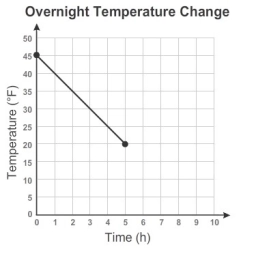# At noon 3

At noon, the temperature was 45˚ F. For the next ten hours, the temperature fell at a steady rate. The graph represents the temperature every hour since noon. At 5:00 temperature was 20°F. At what rate did the temperature fall?

r =  -5 °F/h

### Step-by-step explanation:Did you find an error or inaccuracy? Feel free to write us. Thank you!

Tips for related online calculators
The line slope calculator is helpful for basic calculations in analytic geometry. The coordinates of two points in the plane calculate slope, normal and parametric line equation(s), slope, directional angle, direction vector, the length of the segment, intersections of the coordinate axes, etc.
Do you want to convert time units like minutes to seconds?